Continuous not implies differentiable

This article gives the statement and possibly, proof, of a non-implication relation between two function properties. That is, it states that every function satisfying the first function property (i.e., continuous function) need not satisfy the second function property (i.e., differentiable function)
View a complete list of function property non-implications | View a complete list of function property implications
EXPLORE EXAMPLES YOURSELF: View examples of subgroups satisfying property continuous function but not differentiable function|View examples of subgroups satisfying property continuous function and differentiable function

Statement

It is possible to have a function$f: \R \to \R$ that is a continuous function everywhere but is not a differentiable function everywhere, i.e., it has points where it is not differentiable.

Further, it is possible to construct an example where the function does not have a well-defined left-hand derivative or right-hand derivative.

Proof

Example where one-sided derivatives exist and are not equal

A piecewise definition by interval can be used to construct examples (see differentiation rule for piecewise definition by interval). The simplest example is the absolute value function:$|x| := \left\lbrace \begin{array}{rl}x, & x \ge 0 \\ -x, & x < 0 \\\end{array} \right.$

The function is continuous at 0. The left-hand derivative at 0 is -1 and the right-hand derivative is 1. These are not equal, so the function is not differentiable at 0.

Intuitively, the function is continuous at the point 0 where its definition changes, but it is not differentiable because it turns sharply (from moving down to moving up). There is no well-defined tangent line at (0,0) where it turns.

Example where no one-sided derivatives exist

Consider the function: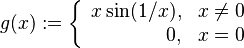$g(x) := \left\lbrace\begin{array}{rl} x \sin(1/x), & x \ne 0 \\ 0, & x = 0 \\\end{array}\right.$

The expression for the function is continuous and differentiable for$x \ne 0$. The expression does not extend to$x = 0$, so we need to calculate the limit at 0 manually to determine if it is continuous and differentiable.

Proof of continuity at 0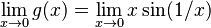$\lim_{x \to 0} g(x) = \lim_{x \to 0} x \sin (1/x)$

We have a bound on absolute value: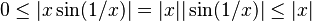$0 \le |x \sin (1/x)| = |x||\sin(1/x)| \le |x|$

Thus:$-|x| \le x\sin (1/x) \le |x|$

Both the leftmost and rightmost expressions approach 0 as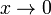$x \to 0$, so by the sandwich theorem, we get:$\lim_{x \to 0} x \sin (1/x) = 0$

Proof of non-differentiability at 0

The derivative at zero is the limit: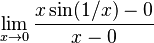$\lim_{x \to 0} \frac{x \sin (1/x) - 0}{x - 0}$

This works out to:$\lim_{x \to 0} \sin(1/x)$

The sine of reciprocal function does not have a limit as$x \to 0$, so this expression is not defined. In fact, even restricting to a one-sided limit gives an undefined expression (for more on this, see the explanation at intermediate value property not implies continuous).